# How to Calculate and Solve for Road Bank Angle, Velocity and Radius of a Body in Motion of Circular Path | The Calculator Encyclopedia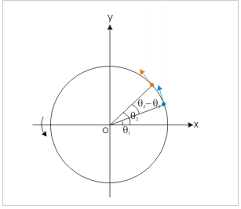The image represents road bank angle in circular motion.

To compute for the road bank angle, three essential parameters are needed and these parameters are velocity (v), acceleration due to gravity (g) and radius (r).

The formula for calculating the road bank angle;

θ = tan-1( / gr)

Where;
v = Velocity
g = Acceleration due to Gravity

Let’s solve an example;
Find the road bank angle where the acceleration due to gravity is 9.8, velocity is 35 and radius is 18.

This implies that;
v = Velocity = 35
g = Acceleration due to Gravity = 9.8

θ = tan-1( / gr)
θ = tan-1(35² / (9.8)(18))
θ = tan-1(1225 / 176.4)
θ = tan-1(6.94)
θ = 81.81°

Therefore, the road bank angle is 81.81°.

Calculating the Velocity when Road Bank Angle, Acceleration due to Gravity and Radius is Given.

v = √gr.tan θ

Where;
v = Velocity
g = Acceleration due to Gravity

Let’s solve an example;
Given that the road bank angle is 50, radius is 15 and acceleration due to gravity is 9.8. Find the velocity?

This implies that;
θ = Road Bank Angle = 50
g = Acceleration due to Gravity = 9.8

v = √gr.tan θ
v = √(9.8 x 15)(tan 50)
v = √(147)(1.1917)
v = √175.1799
v = 13.235

Therefore, the velocity is 13.235.

Calculating the Radius when Road Bank Angle, Acceleration due to Gravity and Velocity is Given.

r = v2 / g.tanθ

Where;
v = Velocity
g = Acceleration due to Gravity

Let’s solve an example;
Given that the road bank angle is 70, velocity is 30 and acceleration due to gravity is 9.8. Find the radius?

This implies that;
θ = Road Bank Angle = 70
g = Acceleration due to Gravity = 9.8
v = Velocity = 30

r = v2 / g.tanθ
r = 302 / 9.8 x tan70
r = 900 / 9.8 x 2.74
r = 900 / 26.852
r = 35.517

Nickzom Calculator – The Calculator Encyclopedia is capable of calculating the road bank angle.

To get the answer and workings of the road bank angle using the Nickzom Calculator – The Calculator Encyclopedia. First, you need to obtain the app.

You can get this app via any of these means:

To get access to the professional version via web, you need to register and subscribe for NGN 1,500 per annum to have utter access to all functionalities.
You can also try the demo version via https://www.nickzom.org/calculator

Once, you have obtained the calculator encyclopedia app, proceed to the Calculator Map, then click on Mechanics under Engineering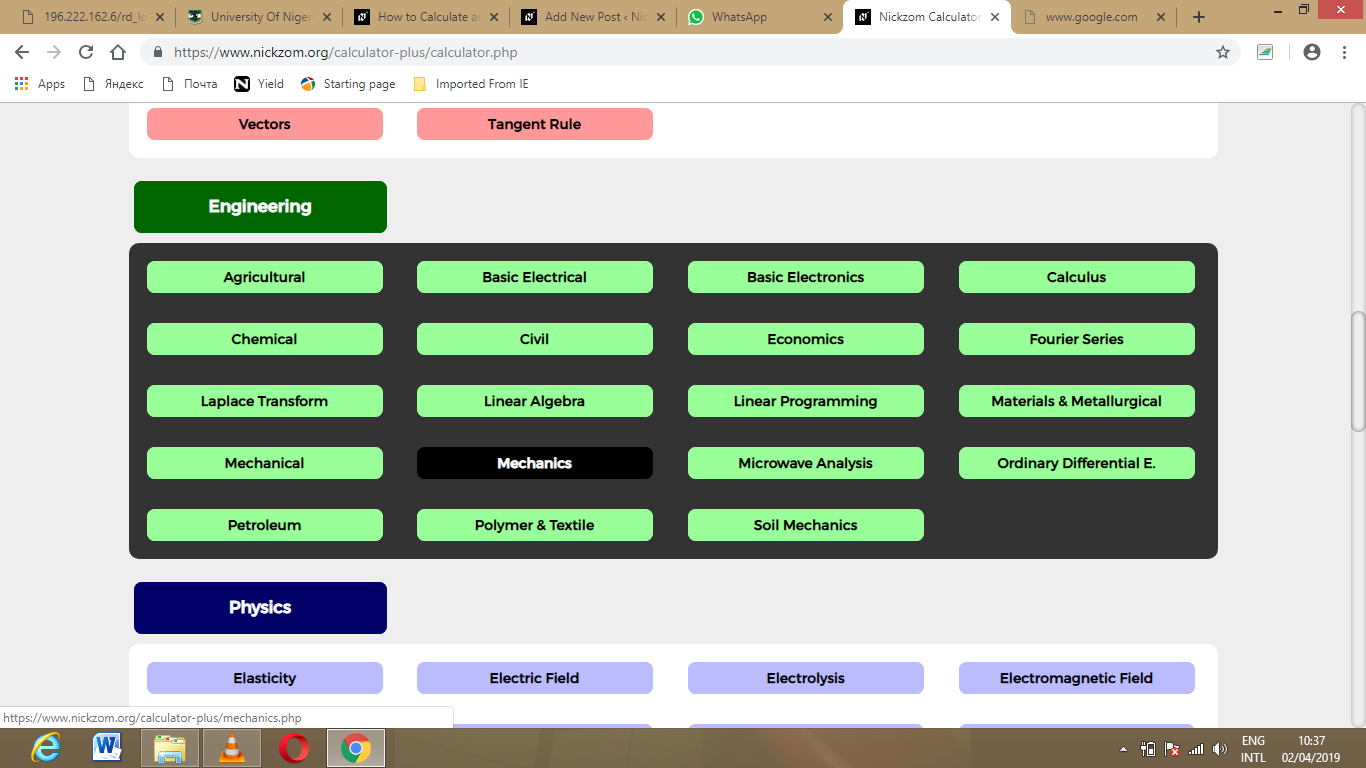Now, Click on Motion of Circular Path under Mechanics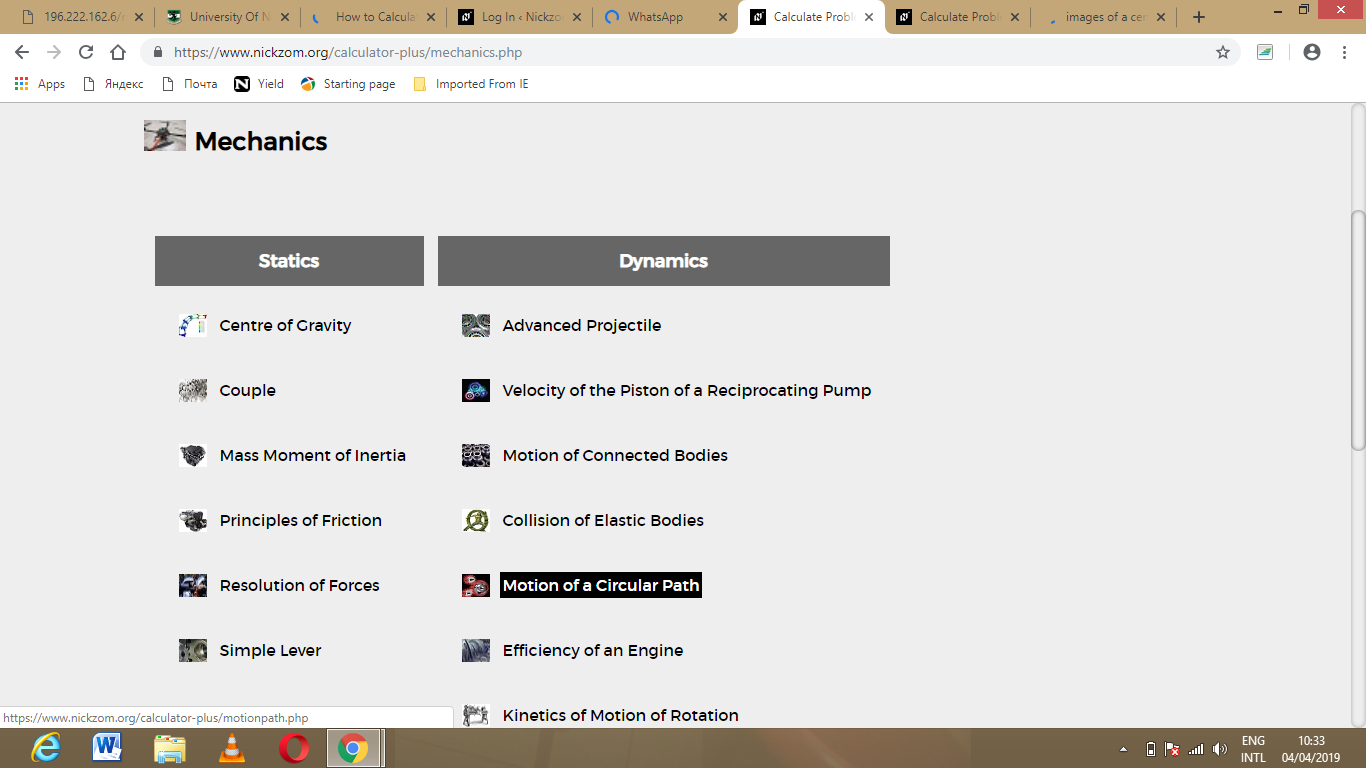Click on Road Bank Angle under Motion of Circular Path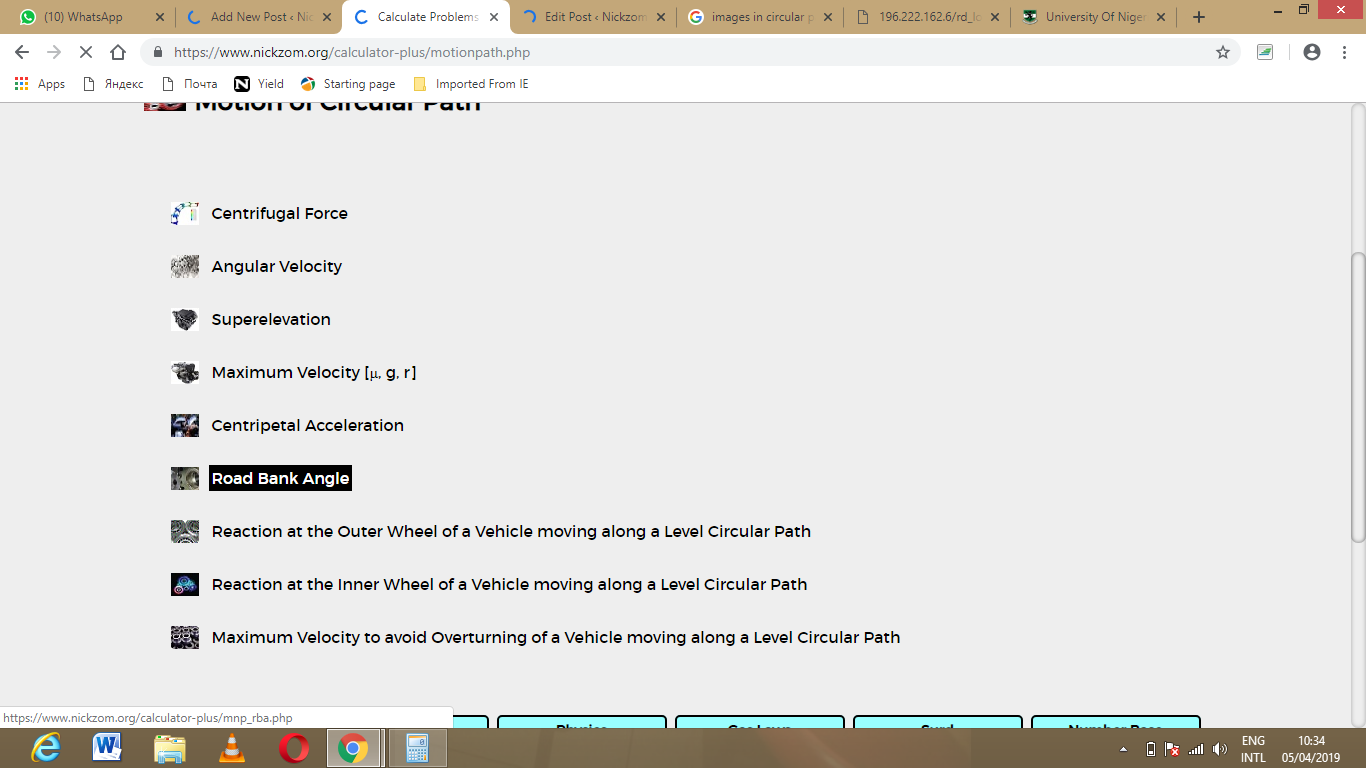The screenshot below displays the page or activity to enter your value, to get the answer for the road bank angle according to the respective parameter which are the velocity (v), acceleration due to gravity (g) and radius (r).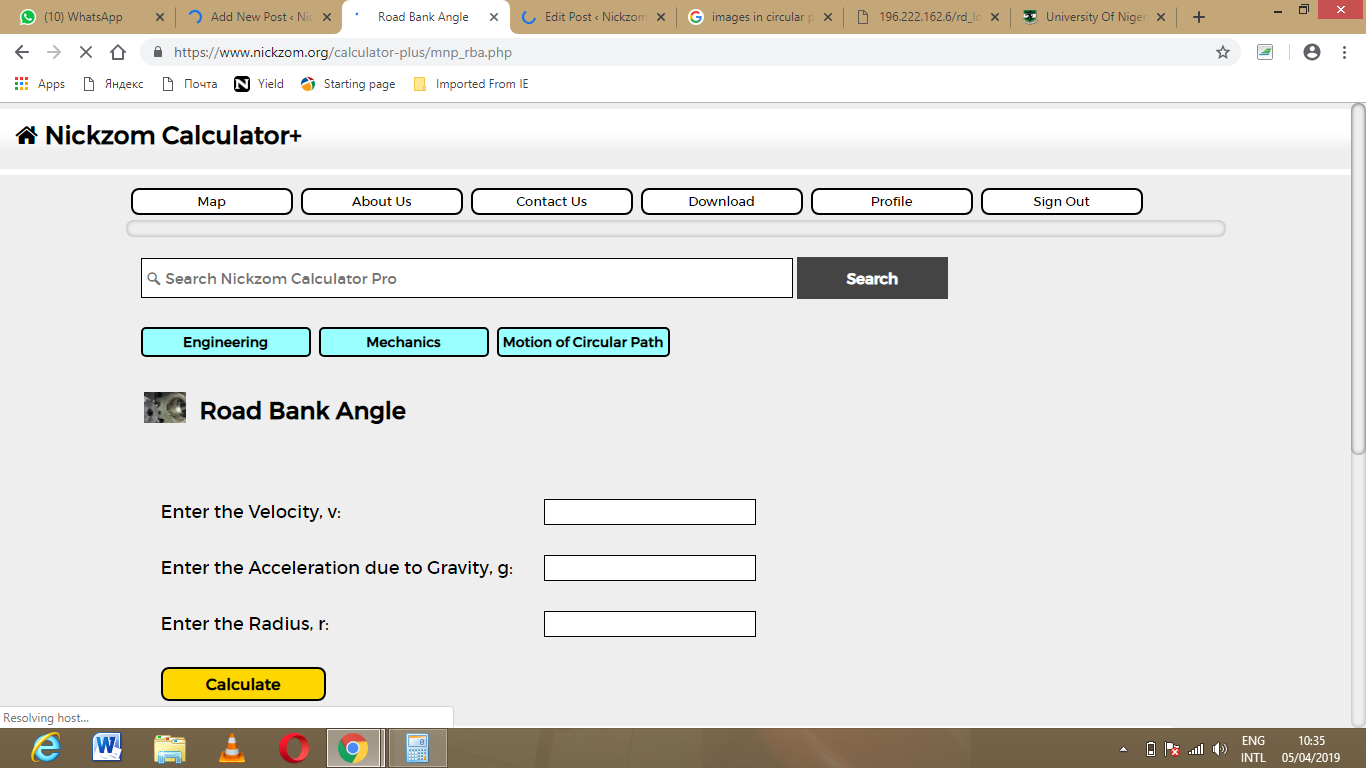Now, enter the value appropriately and accordingly for the parameter as required by the velocity (v) is 35, acceleration due to gravity (g) is 9.8 and radius (r) is 18.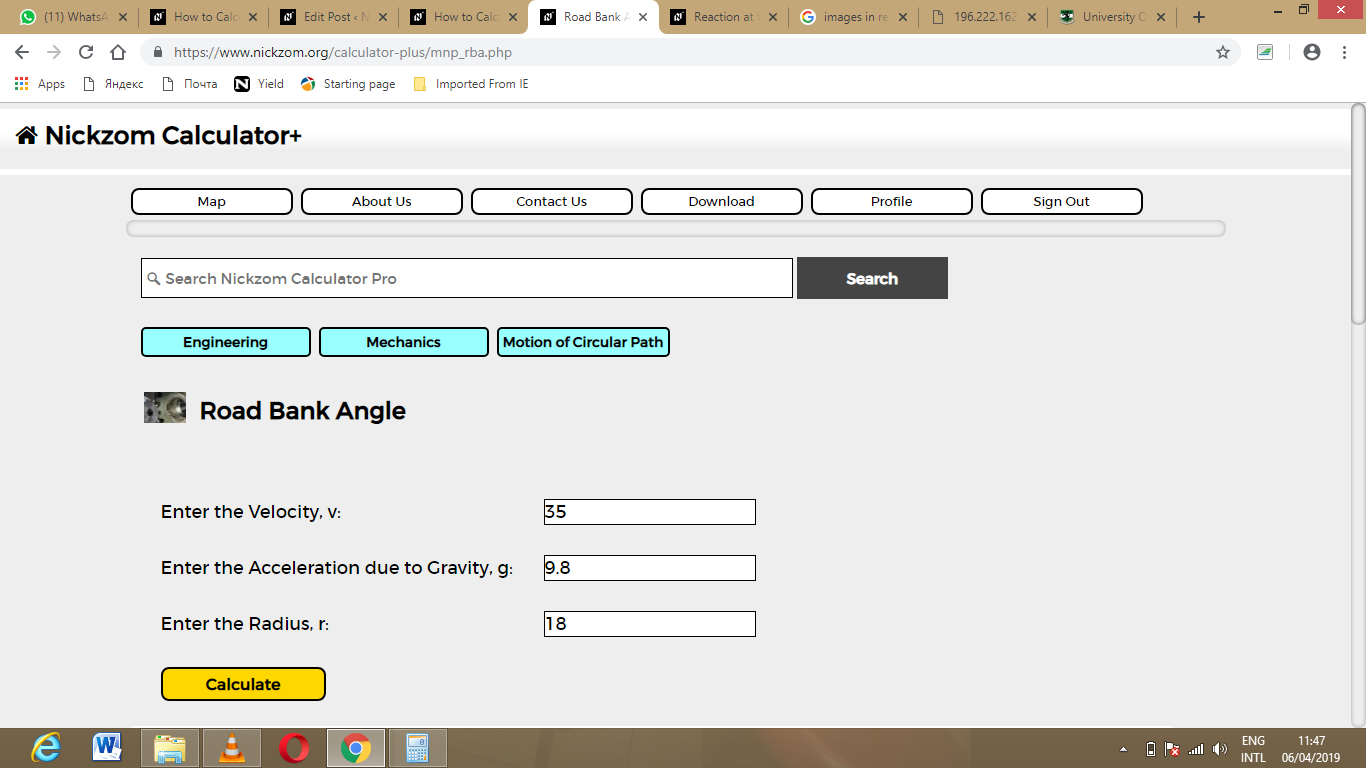Finally, Click on Calculate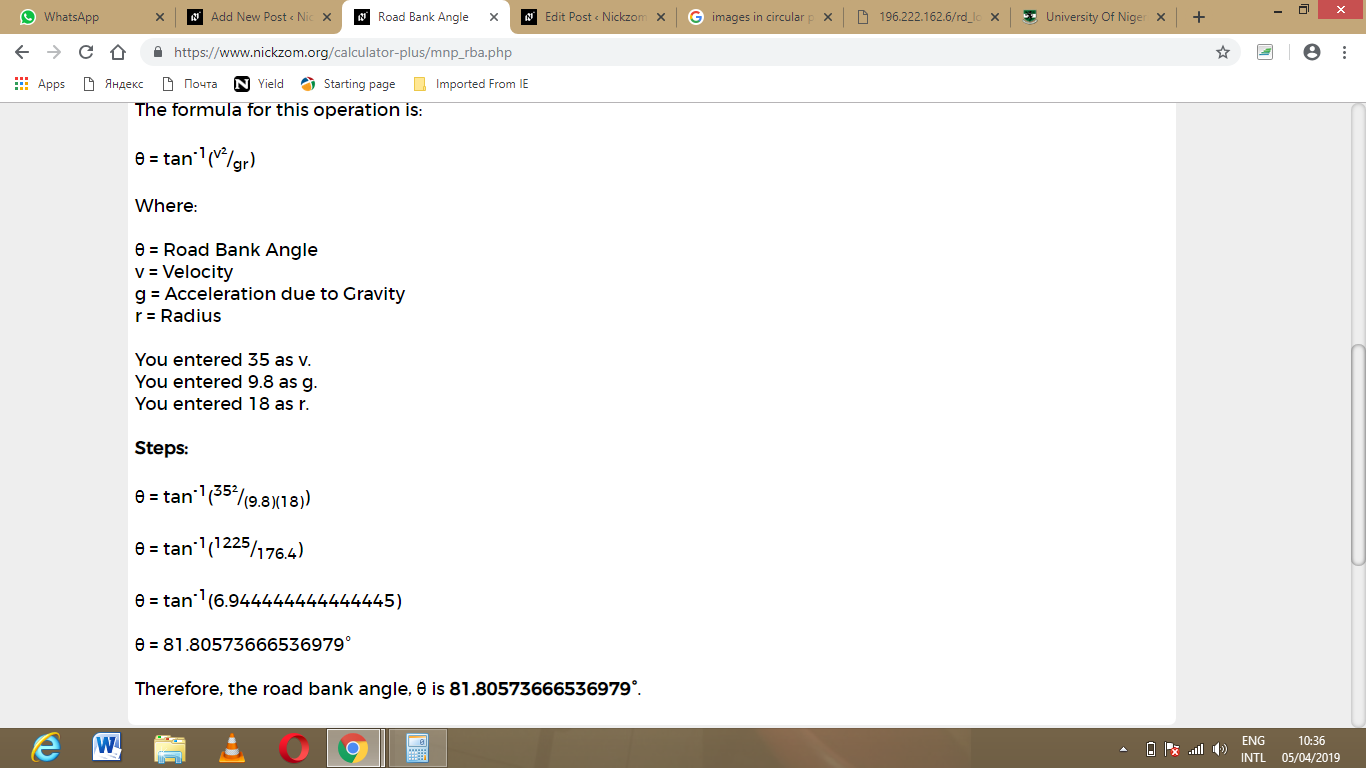As you can see from the screenshot above, Nickzom Calculator – The Calculator Encyclopedia solves for the road bank angle and presents the formula, workings and steps too.